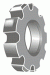I have forgotten

•http://facebook.com/
•https://www.google.com/accounts/o8/id
•https://me.yahoo.com

# Image incomplete with formula \bar{(x+y).\bar{z}}=z+(\bar{x}+\bar{y})28 Aug 17, 6:49AM
(2 replies)
Image incomplete with formula \bar{(x+y).\bar{z}}=z+(\bar{x}+\bar{y})
Hi,

when I render the formula
\bar{(x+y).\bar{z}}=z+(\bar{x}+\bar{y})
the image is incomplete.

When do you think you'll be able to correct the render of this formula ?

Best regards

Hubert Borderiou28 Aug 17, 11:49AM
Actually the fault is with mimetex. bar is and should always be a single character width.

What you want to use is \overline, as in:
\overline{(x+y).\bar{z}}=z+(\bar{x}+\bar{y})
which gives:29 Aug 17, 2:05AM